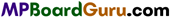These MP Board Class 11th Chemistry Notes for Chapter 5 States of Matter help students to get a brief overview of all the concepts.

## MP Board Class 11th Chemistry Notes Chapter 5 States of Matter

→ Three states of matter are solid, liquid and gas. These states are interconvertible by the effect of temperature and pressure.

→ Attractive force between the molecules is known as intermolecular force.

→ In crystalline solid, molecules are arranged in a definite order.

→ Molecules in gas are arranged in an indefinite order.

→ At STP/NTP, T = 273.15 K, P = 1 atm = 101.325 kPa, V = 22.4 L mol-1.→ 1 Pascal (pa) = 1 Nm-2, 1 atmospheres (atm) = 101325 x 105 Nm-2.

→ Boyle’s law : At constant tçmperature, the volume of definite mass of gas is inversely proportional to pressure (P).
V ∝ $$\frac{1}{\mathrm{P}}$$ or PV = Constant (At constant T) or P1V1 = P2V2

→ Charles’ law: At constant pressure, the volume of a given mass of a gas is directly proportional to absolute temperature.
$$\frac{V_{1}}{T_{1}}=\frac{V_{2}}{T_{2}}$$ (At constant P)

→ Gay Lussac’s law : Whenever gases combine, they combine in simple ratio of their volumes, if the products are in gaseous state, then their volume is also in simple ratio of the reacting gases.
P ∝ T (At Constant V)

→ Avogadro’s law : Equal volume of aH gases, under similar conditions of temperature and pressure contain equal number of molecules.
V ∝ n (At constant temperature and pressure)

→ Ideal gas equation: PV = nRT or P = $$\frac{n \mathrm{RT}}{\mathrm{V}}=\frac{m \mathrm{RT}}{\mathrm{MV}}=\frac{d \mathrm{RT}}{\mathrm{M}}$$
Where d= Density = gL1
PV = RT if n = 1
Where R = Gas constant.

→ Graham’s law of diffusion : At constant temperature and pressure, rate of diffusion of gases is inversely proportional to the square root of their densities.
$$r \propto \sqrt{\frac{1}{d}} \text { or } \frac{d_{2}}{d_{1}}$$(i) Under similar pressure
$$\frac{r_{1}}{r_{2}}=\sqrt{\frac{d_{1}}{d_{2}}}=\sqrt{\frac{\mathrm{M}_{2}}{\mathrm{M}_{1}}}=\frac{\mathrm{V}_{\mathrm{l}} t_{2}}{\mathrm{~V}_{2} t_{1}}$$

Where, d = Vapour density, M = molar mass of gas, V = volume of gas diffused, t = time
required in difusion.

(ii) Diffusion at different pressure:

$$\frac{r_{1}}{r_{2}}=\frac{P_{1}}{P_{2}} \sqrt{\frac{M_{2}}{M_{1}}}=\frac{P_{1}}{P_{2}} \sqrt{\frac{d_{2}}{d_{1}}}$$

→ Kinetic theory: PV = $$\frac{1}{2}$$mu2
Where, u = square root velocity,
KE = $$\frac{3}{2}$$nRT

Average kinetic energy KE. =$$\frac{3}{2} \frac{\mathrm{RT}}{\mathrm{N}_{0}}=\frac{3}{2}$$KT where, K = $$\frac{\mathbf{R}}{\mathrm{N}_{0}}$$ = Boltzmann constant.

→ Dalton’s law of partial pressure: At constant temperature the total pressure of a mixture of
gases is equal to the sum of their partial pressures (P = p1 + p2 + p3 +…………………. ).
P1 = Ptotal. X1
Where, P1, P2 are partial pressure,
X1 = Mole fraction
Pdry gas = Ptotal = Aqueous tension.

→ Kinetic gas equation : PV = $$\frac{1}{3}$$mnv2→ Root Mean Square velocity : R.M.S. velocity is the square root of the average mean of velocities of n moles present in a gas.

→ Average velocity : It is the mathematical mean of velocities of all gas molecules.

→ Ideal gas : Gas which obey gas equation at all temperature and pressure.

→ Compressibility factor : Ratio of observed volume to calculated volume is called Com-pressibility factor.
Z = $$\left[\mathrm{P}+\frac{a n^{2}}{\mathrm{~V}^{2}}\right]$$

→ vander Waals equation :
$$\left[\mathrm{P}+\frac{a n^{2}}{\mathrm{~V}^{2}}\right]$$ [V – nb] = nRT

a = unit = atmL2 mol2
b = unit = L mol-1

→ Boiling point: Temperature at which vapour pressure of a liquid becomes equal to atmospheric pressure.

→ Surface tension : It is an important property related to inter-molecular force of liquids. It is the force acting perpendicular to the surface having unit centimetre length. Due to this property, small drops of liquids are spherical in shape. It decreases with increase in temperature.

→ Viscosity : It is the resistance to the flow of liquid or friction in surface of liquids.

F = ηA $$\frac{d u}{d z}$$
Where, $$\frac{d u}{d z}$$ = velocity gradient
η is expressed by the unit poise.
1 poise = dynes/cm2s = 1 g cm-1s-1 = 10-1 kg m-1s-1.→ Critical temperature : Temperature above which a gas cannot be liquefied.

→ Critical pressure : The minimum pressure by which gas can be liquefied is called Critical pressure.

→ Critical volume : Volume of 1 mole gas at the critical temperature and critical pressure is called Critical volume.